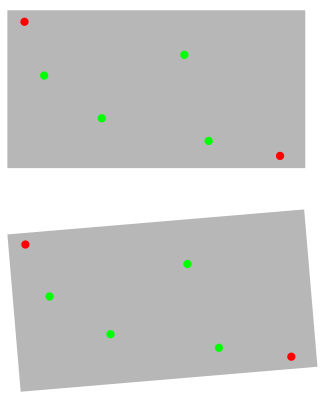QPointF vector mapping with 3*3 matrix

• Hi,
I have a vector of QPointF which contains two registration marks (reg1 and reg2) and a set of points. These point must be placed on a plate which is on the machine bed and always has a slight position error. So with the help of machine vision actual position of registration marks is determined (vreg1 and vreg2).

For example, coordinates are reg1 (2.0,2.0), reg2 (15.0,30.0), and vreg1 (0.6,3.3), vreg2 (8.5,33.1).

I want to map the entire vector according to vreg1 and vreg2.
I tried to use the transform functions (translate and rotate) but of course it doesnt work, i can determine the offsets between reg1/vreg1 and reg2/vreg2, and the coresponding angles, but then Transform::translate and transform::rotate are useless because i dont know the physical rotation origin, nor can i determine the original translation (the translation i measure is the result of original translation + rotation), so the result after translate and rotate never match reality.

It seems that a 3*3 matrix could do that, however i dont understand how i can set it up and use it. From what i understand i should use two matrixes, one with reg1/vreg1 and another with reg2/vreg2 and then apply these matrixes to the vector.

Below is a diagram to clarify, the top figure correspond to the theorical registration reg1 and reg2 (red dots) and the vector of points to map (green dots). The bottom figure correspond to the measured registrations vreg1 and vreg2 (red dot) and the mapped vector of points (green dots) which i need to figure.• Do you think posting it again will help? Esp. since the other post is only 14 hours old: https://forum.qt.io/topic/114108/rotation-of-a-qvector-of-qpointf-or-qpolygonf-from-two-points-instead-of-angle

-> closed